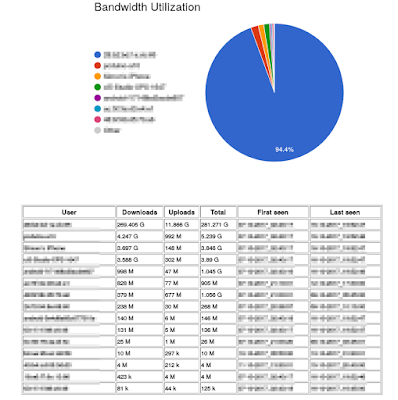## Posts

Showing posts from 2017

### Basics of Integration using Monte Carlo

Recently, someone asked me about Monte Carlo. So, I thought I should write this post to provide a basic introduction of performing integration using Monte Carlo. "Why integration?", you asked. Well, this is because integration is one of the main operations done in computing the posterior probability distributions used in machine learning and probabilistic filtering (e.g. Bayes filter). For example, consider the typical posterior probability expression in the Bayes filtering context, $$p(x_{t} \mid y_{1:t})$$, i.e. the probability of hidden state $$x_{t}$$ given the observed sequence of measurements up to the current time point $$y_{1:t}$$, $$p(x_{t} \mid y_{1:t}) = \frac{p(y_{t} \mid x_{t}) p(x_{t} \mid y_{1:t-1})}{p(y_{t} \mid y_{1:t-1})}$$ The $$p(x_{t} \mid y_{1:t-1})$$ is of particular interest. It can be seen as the prediction of $$x_{t}$$ from previous observations $$y_{1:t-1}$$ and can be expressed as p(x_{t} \mid y_{1:t-1}) = \int p(x_{t} \mid x_{t-1}) p(x_{t-1}

### Having fun with port mirroring, ntopng and traffic shapingOut of interest, I recently managed to set up a monitoring system for my home network. I have been meaning to do this for quite some time already, but never gotten the chance, due to many other commitments. So, here is just a personal note on how I have done so, while having some fun with port mirroring (using iptables/netfilter ), ntopng and traffic shaping (using tc ). First of all, my router (TP-Link TL-WR841N/ND v9) has been set up with OpenWrt, where scripts were written to monitor the relative bandwidth used by each housemate; rules for iptables were written to perform accounting of the cumulative number of bytes sent and received by each host in the network. Basing off wrtbwmon by Peter Bailey , the scripts were further modified to include rules for ip6tables , so that IPv6 traffic could be taken into account. I have also augmented the display of the network statistics using Google Charts API. The result is shown in Figure 1 . Figure 1. A breakdown of the bandwidt